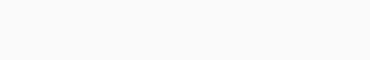# Solved – How to one show a Kmeans solution is unique

Suppose we are given a distribution P and a constant K. We wish to minimize the kmeans objective w.r.t centers \${C1,..Ck}\$:What constraints on \$P\$ are known to imply that the optimal solution is unique (up to reordering of centers)?
Examples of ad hoc methods would also be appreciated.
In particular intereset to me are continuous distributions.

An example for one-dimensional \$P(x)\$ and \$k=2\$ with a unique optimal solution:

\$P(X=1)=0.5\$

\$P(X=-1)=0.5\$

The only optimal centers are \$C1=1, C2=-1\$

Contents

First problem, the solution is not unique.

A simple exemple is \$P(X=-1)=P(X=0)=P(X=1)= frac{1}{3}\$

This gives:Depending on initial conditions. We encounter this problem when we have very particular symmetries. In practice this is very rare, almost impossible.

You may be mixing up this problem with a second one. The convergence of basic k-means is granted to a local minimum of the cost function, not the global minimum. An exemple from Wikipedia:You can adress this problem in many ways: starting near the optimal solution, randomizing the starting points… etc

While the uniqueness of the optimal solution is almost sure, you may end up with many local solutions.

Rate this post